## Example Questions

### Example Question #1 : How To Multiply Integers

A hockey team wants to purchase uniforms for its 26 players, 2 of which are goalies.  It costs $40 for a one-time designing fee. It costs$24 for goalie jerseys and $20 for all other jerseys. How much does it cost to purchase all of the uniforms? Possible Answers:$520

$568$608

$528$664

$568 Explanation: There are 24 normal players and 2 goalies. Thus we can represent the sum in the equation 24($20) + 2($24) +$40 = $568. ### Example Question #2 : How To Multiply Integers Sam is paid$15 per hour for laying bricks. If he lays 3,000 bricks at a rate of 5 bricks per minute, how much will he be paid?

$9,000$200
$150$300
$1,500 Correct answer:$150
Explanation:

First we need to convert some units. If Sam lays 5 bricks per minute, then multiply that figure by 60 to find that he lays 300 bricks per hour. 3,000 bricks divided by 300 bricks/hour means he worked for 10 hours. Therefore, when we mutiply $15/hour by 10 hours, we see he makes$150.

### Example Question #3 : How To Multiply Integers

Joey is beginning to invest in the stock market. He purchased 150 shares of a cell phone company.  The company pays a dividend of $2.55 per share. What is his total dividend? Possible Answers:$451.75

$280.25$301.25

$255.00$382.50

$382.50 Explanation: The words "per share" clues us into a multiplication problem, 150 * 2.55 = 382.50 ### Example Question #4 : How To Multiply Integers George is buying his first car. He has$500 for a downpayment and can afford monthly payments of $175 for three years. What is the most expensive car he can purchase? Possible Answers:$8,000

$7.500$6,800

$4,750$5,250

$6,800 Explanation: The most expensive car he can purchase is given by the total of all monthly payments and the down payment:$175 per month * 12 months per year * 3 years + $500 down =$6,800

### Example Question #5 : How To Multiply Integers

Given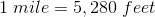, convert 60 miles per hour to approximate feet per second.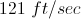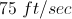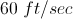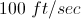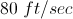Explanation:

Using the factor-label method we get: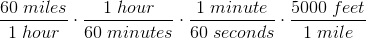approximately equals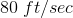The units are treated like numbers and are cancelled when they are in both the  numerator and denominator.

### Example Question #6 : How To Multiply Integers

Which of the following expressions must be an even integer if x is an integer?

x + 3

x6

3x

x/8

x6

Explanation:

An integer raised to an even power always yields an even integer. All of the other expressions could yield odd integers.

### Example Question #7 : How To Multiply Integers

Each variable f, g, h, i, and j represents a positive real number. Considering the equations below, which of the four variables g, h, i, or j necesarily has the greatest value?

1.75g = f

1.69h = f

1.23i = f

0.46j = f

g

h

j

i

j

Explanation:

All four variables are positive real numbers. Each variable g, h, i, and j are multiplied by differing values to obtain the same value f. Since j is multiplied by the smallest value to obtain f it must be the greatest value. In this manner we could determine that g is necesarily the smallest value.

### Example Question #8 : How To Multiply Integers

If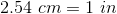, approximately how many cm are in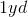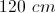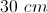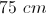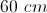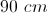Explanation:

Use the factor-label method for conversion: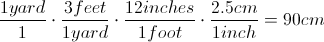The units are considered like numbers and can be cancelled out of both the numerator and the denominator.

### Example Question #9 : How To Multiply Integers

Jordan is packing his lunch, and has decided to bring one piece of fruit, one vegetable, one type of sandwich, and one drink. How many different combinations of lunch can Jordan pack?

 Fruit Vegetable Sandwich Drink Apple Carrot Turkey Milk Banana Celery Chicken Water Orange Veggie

36

10

12

4

14